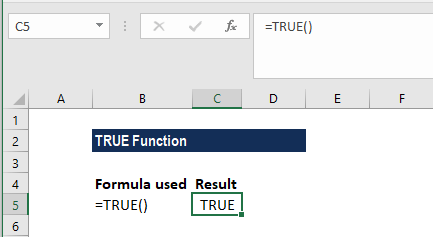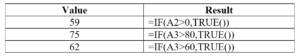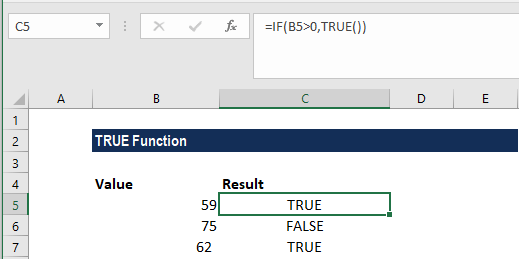# TRUE Function

Returns the logical value TRUE

## What is the TRUE Function?

The TRUE function is categorized under Excel Logical functions. It will return the logical value of TRUE. The function is equivalent to using the Boolean value TRUE directly in a formula.

In financial analysis, the TRUE function is often used with other logical functions such as IF, ERROR, etc. It is related to the FALSE function.

### Formula

=TRUE()

The TRUE function does not require a parameter or argument.

### How to use the TRUE Function in Excel?

As a worksheet function, the TRUE function can be entered as part of a formula in a cell. To understand the uses of the function, let us consider a few examples:

#### Example 1

If we type =TRUE() in a cell, we get the result as TRUE.One can also enter TRUE function directly into a cell. Even if we do not add the parentheses, it will return the Boolean value TRUE.

#### Example 2

Now, let’s consider another example. Using the formulas below, the TRUE function will return the Boolean value TRUE.

The data and formula used are:We get the results below:We can also enter the value TRUE directly into cells and formulas without using the function. For example:

=IF(A1=1, TRUE)

If the condition is met in this example, Excel returns TRUE. If the condition is not met, Excel returns FALSE. We can use either of the formulas:

=IF(A1=1,TRUE())  or

=IF(A1=1,TRUE)

### A few things to remember about the Function

1. The function is provided for compatibility with other spreadsheet applications; it may not be needed in standard situations.
2. If we want to enter TRUE, or if we want to provide TRUE as a result in a formula, we can just enter the word TRUE directly into a cell or formula and Excel will interpret it as the logical value TRUE. For example:

=IF(B1<0, TRUE())

=IF(B1<0, TRUE)

1. We also need to remember that logical expressions themselves will automatically generate TRUE and FALSE results.
2. This function was introduced in MS Excel 2007.

Thanks for reading CFI’s guide to important Excel functions! By taking the time to learn and master these functions, you’ll significantly speed up your financial analysis. To learn more, check out these additional CFI resources:

• Excel Functions for Finance